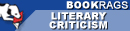# Philosophiae Naturalis Principia Mathematica | Criticism

This literature criticism consists of approximately 29 pages of analysis & critique of Philosophiae Naturalis Principia Mathematica.
 This section contains 8,593 words (approx. 29 pages at 300 words per page) View a FREE sampleSOURCE: "Newton's Mathematical Way," in Journal of the History of Ideas, Vol. XII, No. 1, January 1951, pp. 90-110.

In the following essay, Strong analyzes Newton's method for "mathematically determining" natural phenomena. Aspects of this mathematical procedure, Strong states, include experimental investigation, demonstration from principles, and an emphasis on measurement.

The task of this historical essay is to make out the procedure which Newton designates as a "mathematical way" to be followed in physical science—a way of "mathematically determining all kinds of phenomena."1 This procedure with respect to the role of measurement in experimental inquiry can be called mathematical experimentalism and, with respect to reasoning from principles, mathematical demonstration. Pemberton, the editor of the third edition of the Principia and an expositor of Newton's science, reiterates a position taken by Newton when he states. "The proofs in natural philosophy cannot be so absolutely conclusive, as in the mathematics. For...

 This section contains 8,593 words (approx. 29 pages at 300 words per page) View a FREE sample